# 苏教版数学六年级下册60集合60套试题小升初经典试题附答案 (36)

2020-06-03
15
0
79 KB
10学贝纠错有礼温馨提示：部分包含数学公式或PPT动画的文件，查看预览时可能会显示错乱或异常，文件下载后无此问题，请放心下载。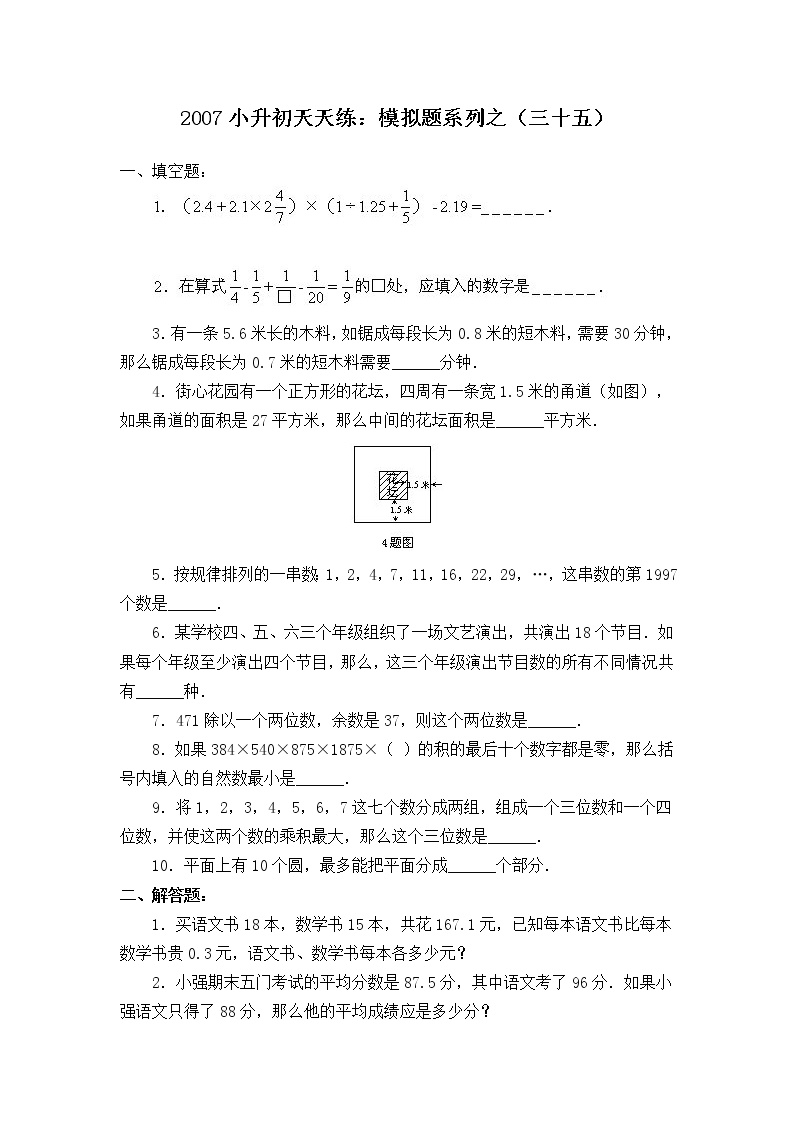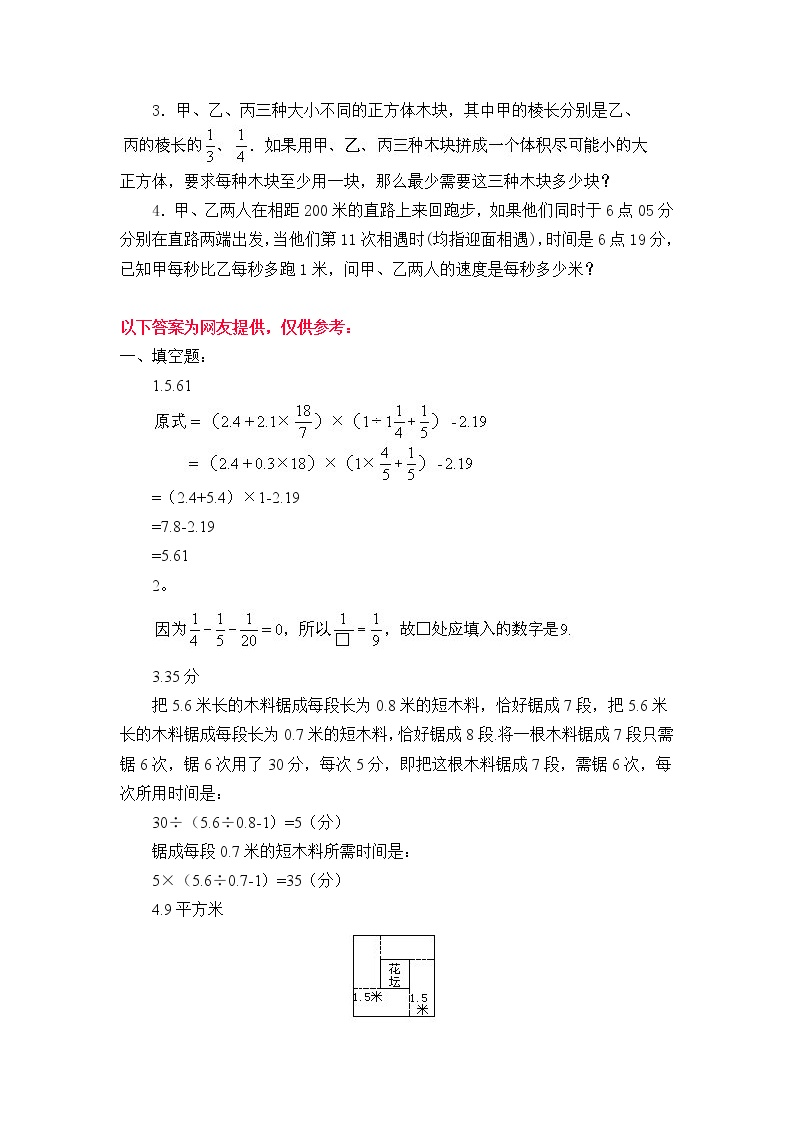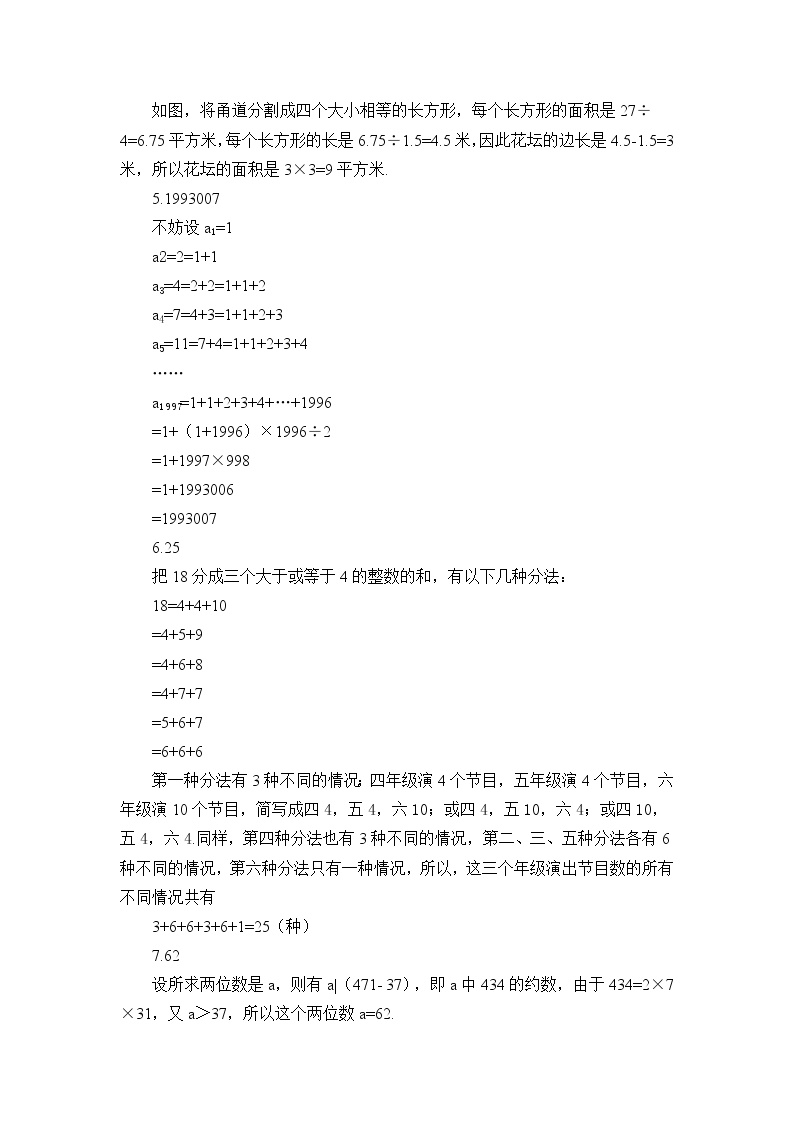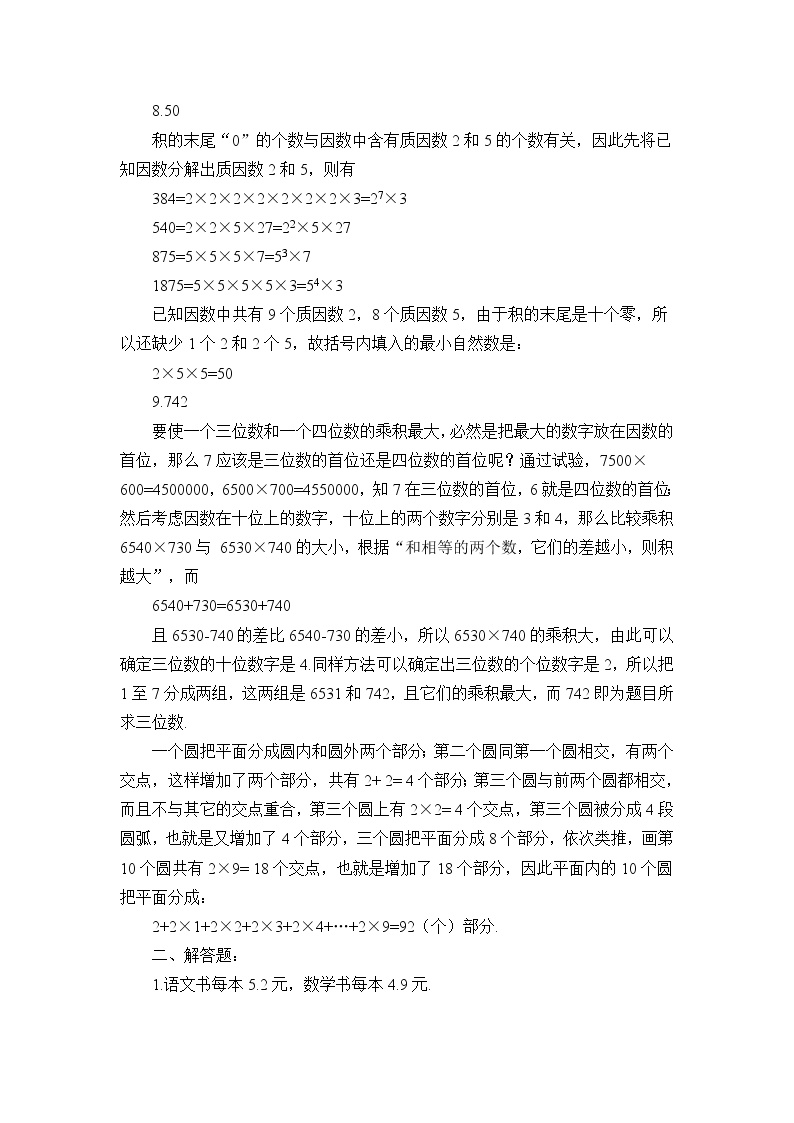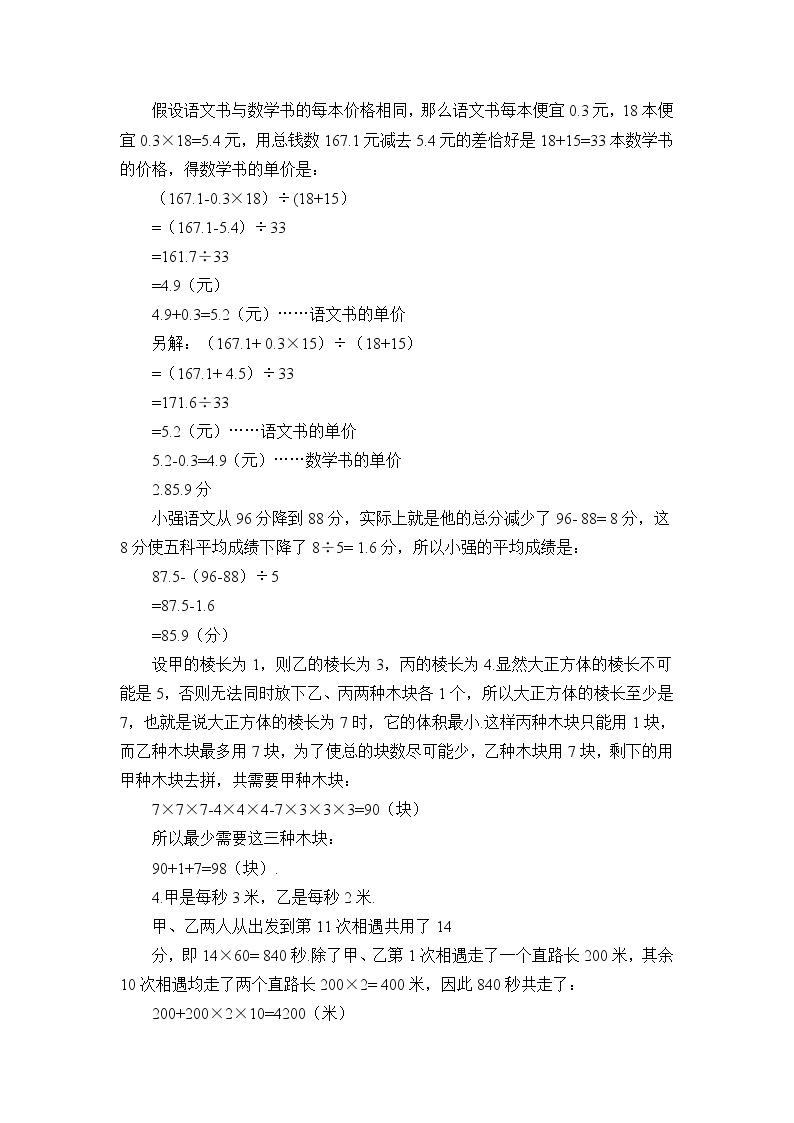2007小升初天天练：模拟题系列之（三十五）

3．有一条5.6米长的木料，如锯成每段长为0.8米的短木料，需要30分钟，那么锯成每段长为0.7米的短木料需要______分钟．

4．街心花园有一个正方形的花坛，四周有一条宽1.5米的甬道（如图），如果甬道的面积是27平方米，那么中间的花坛面积是______平方米．

5．按规律排列的一串数：1，2，4，7，11，16，22，29，…，这串数的第1997个数是______．

6．某学校四、五、六三个年级组织了一场文艺演出，共演出18个节目．如果每个年级至少演出四个节目，那么，这三个年级演出节目数的所有不同情况共有______种．

7．471除以一个两位数，余数是37，则这个两位数是______．

8．如果384×540×875×1875×（ ）的积的最后十个数字都是零，那么括号内填入的自然数最小是______．

9．将1，2，3，4，5，6，7这七个数分成两组，组成一个三位数和一个四位数，并使这两个数的乘积最大，那么这个三位数是______．

10．平面上有10个圆，最多能把平面分成______个部分．

1．买语文书18本，数学书15本，共花167.1元，已知每本语文书比每本数学书贵0.3元，语文书、数学书每本各多少元？

2．小强期末五门考试的平均分数是87.5分，其中语文考了96分．如果小强语文只得了88分，那么他的平均成绩应是多少分？

3．甲、乙、丙三种大小不同的正方体木块，其中甲的棱长分别是乙、

4．甲、乙两人在相距200米的直路上来回跑步，如果他们同时于6点05分分别在直路两端出发，当他们第11次相遇时(均指迎面相遇)，时间是6点19分，已知甲每秒比乙每秒多跑1米，问甲、乙两人的速度是每秒多少米？

1.5.61

=2.4+5.4×1-2.19

=7.8-2.19

=5.61

2

3.35

把5.6米长的木料锯成每段长为0.8米的短木料，恰好锯成7段，把5.6米长的木料锯成每段长为0.7米的短木料，恰好锯成8.将一根木料锯成7段只需锯6次，锯6次用了30分，每次5分，即把这根木料锯成7段，需锯6次，每次所用时间是：

30÷5.6÷0.8-1=5（分）

锯成每段0.7米的短木料所需时间是：

5×5.6÷0.7-1=35（分）

4.9平方米

如图，将甬道分割成四个大小相等的长方形，每个长方形的面积是27÷4=6.75平方米，每个长方形的长是6.75÷1.5=4.5米，因此花坛的边长是4.5-1.5=3米，所以花坛的面积是3×3=9平方米.

5.1993007

不妨设a1=1

a2=2=1+1

a3=4=2+2=1+1+2

a4=7=4+3=1+1+2+3

a5=11=7+4=1+1+2+3+4

……

a1997=1+1+2+3+4++1996

=1+1+1996×1996÷2

=1+1997×998

=1+1993006

=1993007

6.25

把18分成三个大于或等于4的整数的和，有以下几种分法：

18=4+4+10

=4+5+9

=4+6+8

=4+7+7

=5+6+7

=6+6+6

第一种分法有3种不同的情况；四年级演4个节目，五年级演4个节目，六年级演10个节目，简写成四4，五4，六10；或四4，五10，六4；或四10，五4，六4.同样，第四种分法也有3种不同的情况，第二、三、五种分法各有6种不同的情况，第六种分法只有一种情况，所以，这三个年级演出节目数的所有不同情况共有

3+6+6+3+6+1=25（种）

7.62

设所求两位数是a，则有a|471- 37），即a434的约数，由于434=2×7×31，又a37，所以这个两位数a=62.

8.50

积的末尾0的个数与因数中含有质因数25的个数有关，因此先将已知因数分解出质因数25，则有

384=2×2×2×2×2×2×2×3=27×3

540=2×2×5×27=22×5×27

875=5×5×5×7=53×7

1875=5×5×5×5×3=54×3

已知因数中共有9个质因数28个质因数5，由于积的末尾是十个零，所以还缺少1225，故括号内填入的最小自然数是：

2×5×5=50

9.742

要使一个三位数和一个四位数的乘积最大，必然是把最大的数字放在因数的首位，那么7应该是三位数的首位还是四位数的首位呢？通过试验，7500×600=45000006500×700=4550000，知7在三位数的首位，6就是四位数的首位；然后考虑因数在十位上的数字，十位上的两个数字分别是34，那么比较乘积6540×7306530×740的大小，根据和相等的两个数，它们的差越小，则积越大，而

6540+730=6530+740

且6530-740的差比6540-730的差小，所以6530×740的乘积大，由此可以确定三位数的十位数字是4.同样方法可以确定出三位数的个位数字是2，所以把17分成两组，这两组是6531742，且它们的乘积最大，而742即为题目所求三位数.

一个圆把平面分成圆内和圆外两个部分；第二个圆同第一个圆相交，有两个交点，这样增加了两个部分，共有2+ 2= 4个部分；第三个圆与前两个圆都相交，而且不与其它的交点重合，第三个圆上有2×2= 4个交点，第三个圆被分成4段圆弧，也就是又增加了4个部分，三个圆把平面分成8个部分，依次类推，画第10个圆共有2×9= 18个交点，也就是增加了18个部分，因此平面内的10个圆把平面分成：

2+2×1+2×2+2×3+2×4++2×9=92（个）部分.

二、解答题：

1.语文书每本5.2元，数学书每本4.9.

假设语文书与数学书的每本价格相同，那么语文书每本便宜0.3元，18本便宜0.3×18=5.4元，用总钱数167.1元减去5.4元的差恰好是18+15=33本数学书的价格，得数学书的单价是：

（167.1-0.3×18÷(18+15

=167.1-5.4÷33

=161.7÷33

=4.9（元）

4.9+0.3=5.2（元）……语文书的单价

另解：（167.1+ 0.3×15÷18+15

=167.1+ 4.5÷33

=171.6÷33

=5.2（元）……语文书的单价

5.2-0.3=4.9（元）……数学书的单价

2.85.9

小强语文从96分降到88分，实际上就是他的总分减少了96- 88= 8分，这8分使五科平均成绩下降了8÷5= 1.6分，所以小强的平均成绩是：

87.5-96-88÷5

=87.5-1.6

=85.9（分）

设甲的棱长为1，则乙的棱长为3，丙的棱长为4.显然大正方体的棱长不可能是5，否则无法同时放下乙、丙两种木块各1个，所以大正方体的棱长至少是7，也就是说大正方体的棱长为7时，它的体积最小.这样丙种木块只能用1块，而乙种木块最多用7块，为了使总的块数尽可能少，乙种木块用7块，剩下的用甲种木块去拼，共需要甲种木块：

7×7×7-4×4×4-7×3×3×3=90（块）

所以最少需要这三种木块：

90+1+7=98（块）.

4.甲是每秒3米，乙是每秒2.

甲、乙两人从出发到第11次相遇共用了14

分，即14×60= 840.除了甲、乙第1次相遇走了一个直路长200米，其余10次相遇均走了两个直路长200×2= 400米，因此840秒共走了：

200+200×2×10=4200（米）

这样得到甲、乙两人速度和是每秒走：

4200÷840=5（米）

又知甲与乙的速度差是每秒1米，由此得甲速度是每秒走：

（5+1÷2=3（米）

乙每秒走：

（5-1÷2=2（米）.